# 'Quick, Draw!' – Classifying Drawings with Python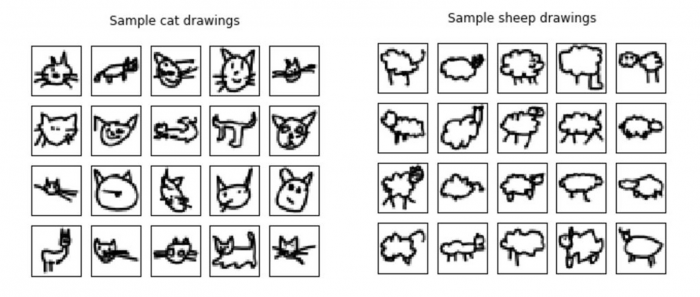Image recognition has been a major challenge in machine learning, and working with large labelled datasets to train your algorithms can be time-consuming. One efficient approach for getting such data is to outsource the work to a large crowd of users. Google uses this approach with the game “Quick, Draw!” to create the world’s largest doodling dataset, which has recently been made publicly available. In this game, you are told what to draw in less than 20 seconds while a neural network is predicting in real time what the drawing represents. Once the prediction is correct, you can't finish your drawing, which results in some drawings looking rather weird (e.g., animals missing limbs). The dataset consists of 50 million drawings spanning 345 categories, including animals, vehicles, instruments and other items. By contrast, the MNIST dataset – also known as the “Hello World” of machine learning – includes no more than 70,000 handwritten digits. Compared with digits, the variability within each category of the “Quick, Draw!” data is much bigger, as there are many more ways to draw a cat than to write the number 8, say.

## A Convolutional Neural Network in Keras Performs Best

With the project summarized below, we aimed to compare different machine learning algorithms from scikit-learn and Keras tasked with classifying drawings made in “Quick, Draw!”. Here we will present the results without providing any code, but you can find our Python code on Github. We used a dataset that had already been preprocessed to a uniform 28x28 pixel image size. Other datasets are available too, including for the original resolution (which varies depending on the device used), timestamps collected during the drawing process, and the country where the drawing was made.

We first tested different binary classification algorithms to distinguish cats from sheep, a task that shouldn’t be too difficult. Figure 1 gives you an idea of what these drawings look like. While there are over 120,000 images per category available, we only used up to 7,500 per category, as training gets very time-consuming with an increasing number of samples.

##### Figure 1: Samples of the drawings made by users of the game “Quick, Draw!”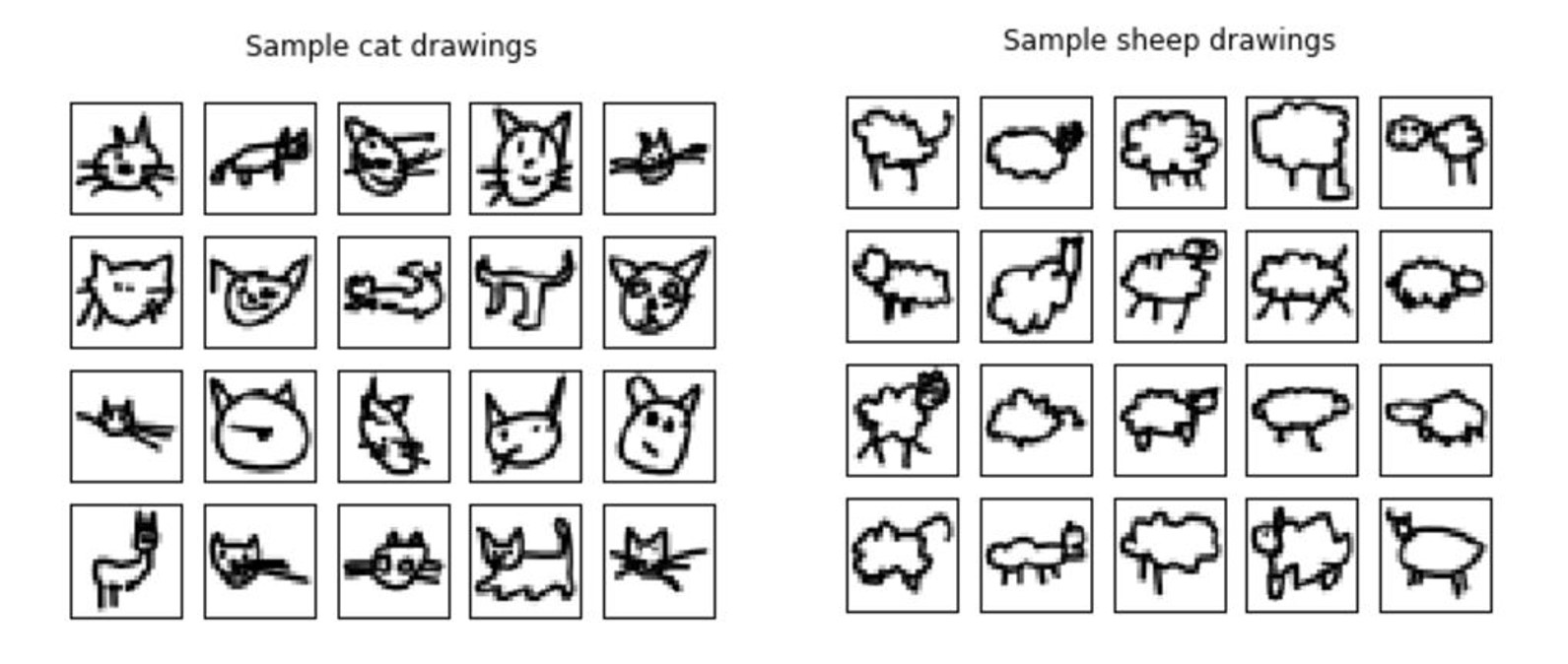We tested the Random Forest, K-Nearest Neighbors (KNN) and Multi-Layer Perceptron (MLP) classifiers in scikit-learn as well as a Convolutional Neural Network (CNN) in Keras. The performance of these algorithms on a test set is shown in Figure 2. As one might expect, the CNN had the highest accuracy by far (up to 96%), but it also required the longest computing time. The KNN classifier came in second, followed by the MLP and Random Forest.

##### Figure 2: Accuracy scores for RF, KNN, MLP and CNN classifiers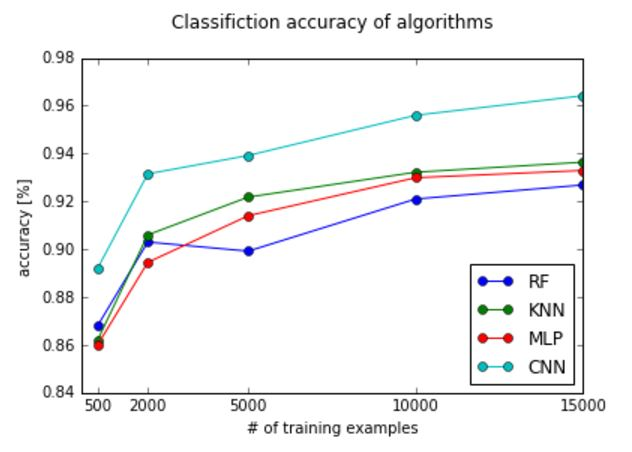## Optimizing the Parameters

The most important parameter for the Random Forest classifier is the number of trees, which is set to 10 by default in scikit-learn. Increasing this number usually achieves a higher accuracy, but computing time also increases significantly. For this task, we found 100 trees to be a sufficient number, as there is hardly any increase in accuracy beyond that.

In the K-Nearest Neighbor classifier, the central parameter is K, the number of neighbors. The default option is 5, which we found to be optimal in this case.

For the Multi-Layer Perceptron (MLP), the structure of the hidden layer(s) is a major point to consider. The default option is one layer of 100 nodes. We tried one or two layers of 784 nodes, which slightly increased accuracy at the cost of a much higher computing time. We chose two layers of 100 nodes as a compromise between accuracy and time use (adding a second layer did not change the accuracy, but it reduced fitting time by half). The difference between learning rates was very small, with the best results using 0.001.

For the Convolutional Neural Network in Keras (using TensorFlow backend), we adapted the architecture from a tutorial by Jason Brownlee. In short, this CNN is composed of the following 9 layers: 1) Convolutional layer with 30 feature maps of size 5×5, 2) Pooling layer taking the max over 2*2 patches, 3) Convolutional layer with 15 feature maps of size 3×3, 4) Pooling layer taking the max over 2*2 patches, 5) Dropout layer with a probability of 20%, 6) Flatten layer, 7) Fully connected layer with 128 neurons and rectifier activation, 8) Fully connected layer with 50 neurons and rectifier activation, 9) Output layer.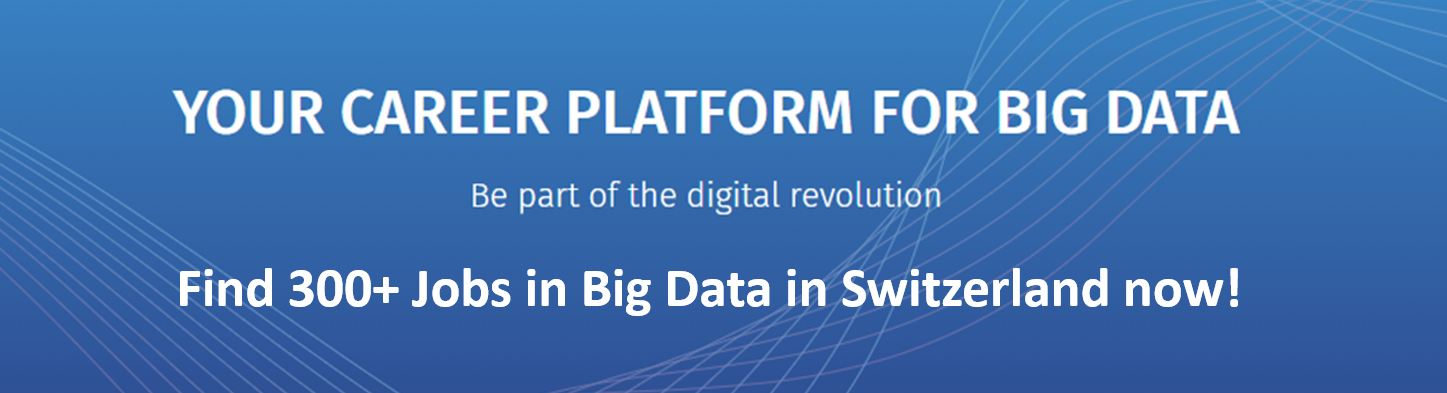## Evaluation of the CNN Classifier

Beside the accuracy score, there are other useful ways to evaluate a classifier. We can get valuable insights by looking at the images that were classified incorrectly. Figure 3 shows some examples for the top performing CNN. Many of these are hard or impossible even for competent humans to classify, but they also include some that seem doable.

##### Figure 3: Examples of images misclassified by the CNN (trained on 15,000 samples)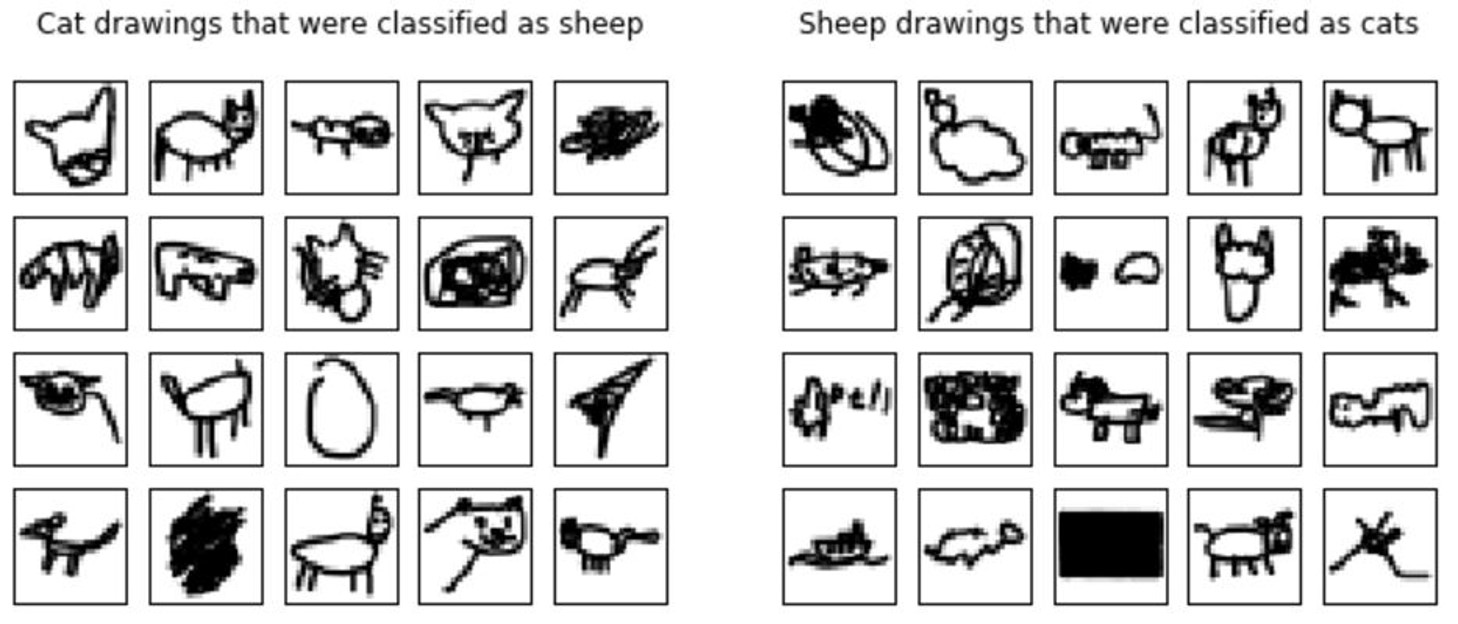To obtain the actual number of images classified incorrectly, we can calculate the confusion matrix (Figure 4). In this example, the decision threshold was set to 0.5, implying that the label with the higher probability was predicted. For other applications, e.g. testing for a disease, a different threshold may be better suited. One metric that considers all possible thresholds is the Area Under the Curve (AUC) score that is calculated from the Receiver Operating Characteristic (ROC) curve. The ROC curve plots the true positive rate (TPR) against the false positive rate (FPR) at various threshold settings (but it doesn’t show which threshold was used for a specific point of the curve). As the name says, the AUC is simply the area under the ROC curve, which would be 1 for a perfect classifier and 0.5 for random guessing. The ROC curve for the CNN is shown in Figure 4, and the associated AUC score is at a very respectable 0.994.

##### Figure 4: Confusion matrix and ROC curve of the CNN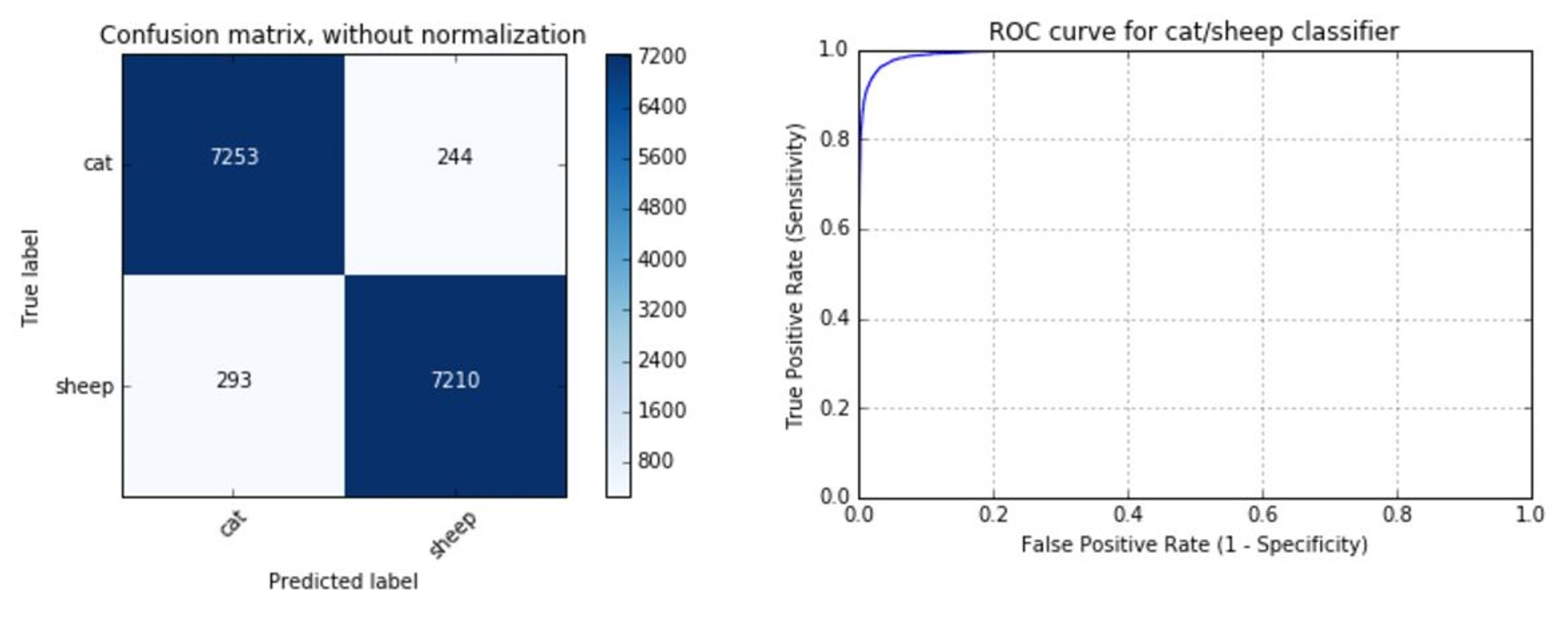## Multi-Class Classification

To make things more challenging, we also tested the algorithms on five different classes (dog, octopus, bee, hedgehog, giraffe), using 2,500 images of each class for training. As expected, we got a similar ranking as before, but the accuracies were lower: 79% for Random Forest, 81% MLP, 82% KNN, and 90% CNN.

Looking at the actual probabilities placed on each class allows a better idea of what the classifier is predicting. Figure 5 shows some examples for the CNN. Most predictions come with a high degree of certainty, which is not surprising given an accuracy of 90%. If the predictions are well calibrated – which they are in this case –, the average certainty of the predicted class must be 90% as well.

##### Figure 5: Some CNN prediction probabilities.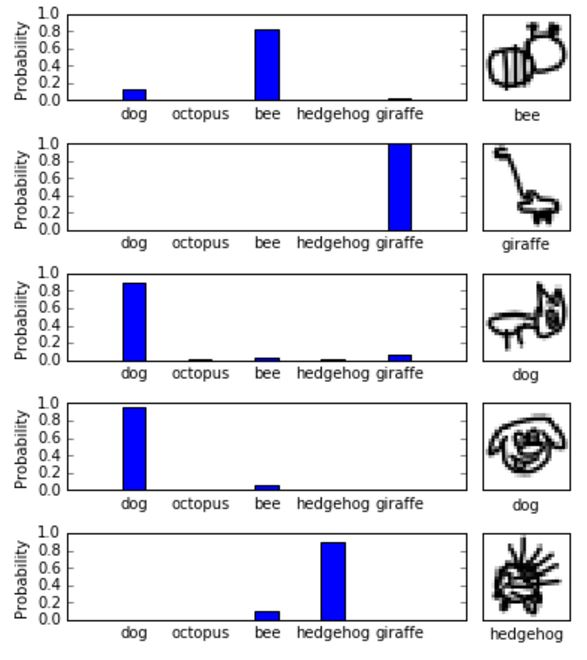Figure 6 shows a few predictions that were incorrect. While these are rather unconventional drawings, competent humans might well recognize them all, or give more accurate probability distributions at least. There certainly is room for improvement, the easiest way being to simply increase the number of training examples (we only used about 2% of the images).

##### Figure 6: Some drawings that were misclassified by the CNN. The true label is shown below the image.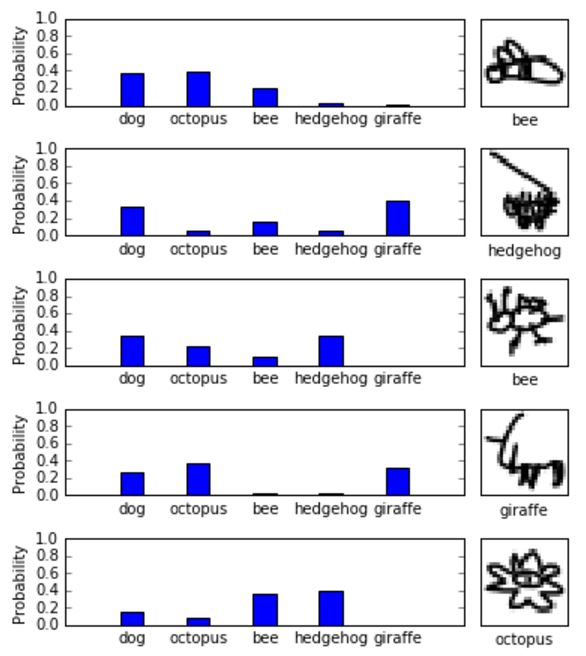In conclusion, what have we learned from the above analysis? If you take a look at the code, you will see that implementing a CNN in Python takes more effort than the regular scikit-learn classifiers do, which comprise just a few lines. The superior accuracy of the CNN makes this investment worthwhile, though. Due to its huge size, the “Quick, Draw!” dataset is very valuable if you’re interested in image recognition and deep learning. We have barely scratched the surface of the dataset here and there remains huge potential for further analysis. To check out the code and some additional analyses, visit our Github page. We’re very happy to receive any feedback and suggestions for improvement by email.

A blog post by David Kradolfer